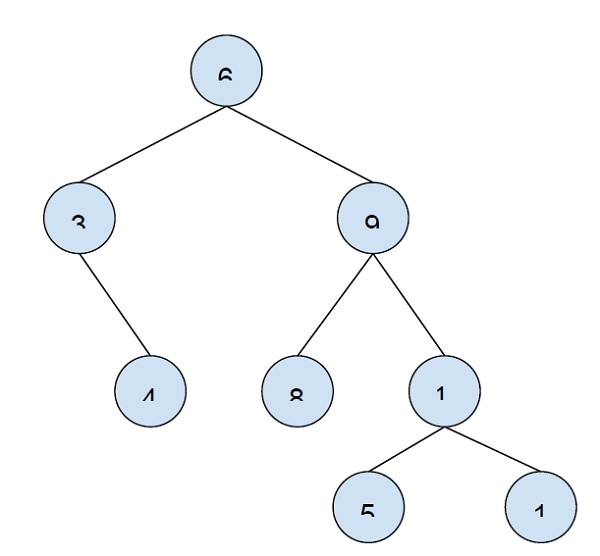# Print all nodes that are at distance k from a leaf node in C++

In this problem, we are given a binary tree and a number K. We have to print all nodes of the tree that are at k distance from the leaf node.

Binary Tree is a special tree whose each node has at max two nodes (one/two/none).

The leaf node of a binary tree is the node at end of the tree.

In this problem, distance from the leaf node is the node at a higher level than the leaf node. Suppose, the node at distance 2 from the leaf node at level 4 will be at level 2.

Let’s take an example to understand the problemK = 2

Output − 6 9.

To solve this problem, we will traverse the tree. All store all parent node sets (also know as ancestor nodes) level by level will the leaf node is reached. Now, we will print the at ancestor k distance away from the leaf node. While traversal marking of visited nodes is important to avoid duplicate and we will use a boolean array for this purpose −

As the code uses only tree traversals the complexity is proportional to n. Time Complexity: O(n)

## Example

Program to illustrate our logic −

#include <iostream>
using namespace std;
#define MAX_HEIGHT 10000
struct Node {
int key;
Node *left, *right;
};
Node* insertNode(int key){
Node* node = new Node;
node->key = key;
node->left = node->right = NULL;
return (node);
}
void nodesKatDistance(Node* node, int path[], bool visited[], int pathLen, int k){
if (node==NULL) return;
path[pathLen] = node->key;
visited[pathLen] = false;
pathLen++;
if (node->left == NULL && node->right == NULL && pathLen-k-1 >= 0 && visited[pathLen-k-1] == false){
cout<<path[pathLen-k-1]<<"\t";
visited[pathLen-k-1] = true;
return;
}
nodesKatDistance(node->left, path, visited, pathLen, k);
nodesKatDistance(node->right, path, visited, pathLen, k);
}
void printNodes(Node* node, int k){
int path[MAX_HEIGHT];
bool visited[MAX_HEIGHT] = {false};
nodesKatDistance(node, path, visited, 0, k);
}
int main(){
Node * root = insertNode(6);
root->left = insertNode(3);
root->right = insertNode(9);
root->left->right = insertNode(4);
root->right->left = insertNode(8);
root->right->right = insertNode(10);
root->right->left->left = insertNode(5);
root->right->left->right = insertNode(1);
int k = 2 cout<<"All nodes at distance "<<k<<" from leaf node are:\n";
printNodes(root, k);
return 0;
}

## Output

All nodes at distance 2 from the leaf node are −

6 9